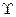Chapter 33, Problem 38PE

Chapter
Section
Textbook Problem

(a) Find the charge, baryon number, strangeness, charm, and bottomness of theparticle from its quark composition.(b) Do the same for theparticle.

To determine

(a)

The charge, baryon number, strangeness, charm and bottomness of the J/ψ particle from

its quark composition

Explanation

Given:

Quarks are fundamental particles.

Calculation:

Quark structure of J/ψ particle is cc¯.

Baryon number of quark c=13 and that of antiquark c¯=13

So, baryon number of J/ψ particle =1313=0

Charge of quark c=+23e and that of antiquark c¯=23e

So, charge of J/ψ particle =23e23e=0

Strangeness number of quark

To determine

(b)

The charge, baryon number, strangeness, charm and bottomness of the Γ particle from

its quark composition

Still sussing out bartleby?

Check out a sample textbook solution.

See a sample solution

The Solution to Your Study Problems

Bartleby provides explanations to thousands of textbook problems written by our experts, many with advanced degrees!

Get Started